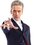# Beginners $A$gebra 4: Index Notation

Index Notation is a simple area of maths and is not just part of algebra. If you have multiple of the same numbers multiplying by each other and the question asks you to put it in index form you don't solve it but what you do is you put the number (Base Number) and the small number to the top right (Power Number*). Here is an example below

2 x 2 x 2 = $2^{3}$

if you see multiple of the same numbers and multiple of another number also like below and you are required to put them in index form DO NOT MIX THEM as this is incorrect.

3 x 3 x 3 x 5 x 5 x 5 x 5 x 5 = 3 x 3 x 3 = $3^{3}$ ; 5 x 5 x 5 x 5 x 5 = $5^{5}$

therefore: 3 x 3 x 3 x 5 x 5 x 5 x 5 x 5 = $3^{3}$ x $5^{5}$

Now we understand the basics of it lets use it in algebra. When beginners hear algebra get involved they think it is very complicated but it is not if you use the same thing as you did before.

a x a x a x b x b x b x b x b = $a^{3}$ x $b^{5}$

More Coming Soon! This will be a question soon but until i have put all the info i am saving it as a draft as a conversation. ETP-5 days

(Note this should not be taken as an official guide and it is recommended you still study further.)Note by Nscs 747
6 months, 2 weeks ago

This discussion board is a place to discuss our Daily Challenges and the math and science related to those challenges. Explanations are more than just a solution — they should explain the steps and thinking strategies that you used to obtain the solution. Comments should further the discussion of math and science.

When posting on Brilliant:

• Use the emojis to react to an explanation, whether you're congratulating a job well done , or just really confused .
• Ask specific questions about the challenge or the steps in somebody's explanation. Well-posed questions can add a lot to the discussion, but posting "I don't understand!" doesn't help anyone.
• Try to contribute something new to the discussion, whether it is an extension, generalization or other idea related to the challenge.

MarkdownAppears as
*italics* or _italics_ italics
**bold** or __bold__ bold
- bulleted- list
• bulleted
• list
1. numbered2. list
1. numbered
2. list
Note: you must add a full line of space before and after lists for them to show up correctly
paragraph 1paragraph 2

paragraph 1

paragraph 2

[example link](https://brilliant.org)example link
> This is a quote
This is a quote
    # I indented these lines
# 4 spaces, and now they show
# up as a code block.

print "hello world"
# I indented these lines
# 4 spaces, and now they show
# up as a code block.

print "hello world"
MathAppears as
Remember to wrap math in $$ ... $$ or $ ... $ to ensure proper formatting.
2 \times 3 $2 \times 3$
2^{34} $2^{34}$
a_{i-1} $a_{i-1}$
\frac{2}{3} $\frac{2}{3}$
\sqrt{2} $\sqrt{2}$
\sum_{i=1}^3 $\sum_{i=1}^3$
\sin \theta $\sin \theta$
\boxed{123} $\boxed{123}$

Sort by:

a * a * a * b * b * b * b * b = x^3 * b^5

smh @NSCS 747

- 6 months, 2 weeks ago

? what?

- 6 months, 2 weeks ago

where the fish did X come from? the actual variable is 'A' 🙄

- 6 months, 2 weeks ago

i was copying and pasting latex from the first one i typed so yea

- 6 months, 2 weeks ago

oh i see it shoot

- 6 months, 2 weeks ago

k good

- 6 months, 2 weeks ago

i was copying and pasting latex cuz i was lazy to type it

- 6 months, 2 weeks ago

You should do parentheses (brackets) next

- 6 months, 1 week ago

for anyone wondering for my next ones i will use brackets i will probably make about 2 more before taking another break for a few weeks

- 5 months, 2 weeks ago